Mathematics is the science that deals with the logic of shape, quantity, and arrangement. Math is all around us, in everything we do. It is the building block for everything in our daily lives, including mobile devices, architecture (ancient and modern), art, money, engineering, and even sports. It represents a high level of abstraction attained by the human mind.

## 10 Indian Inventions on Mathematics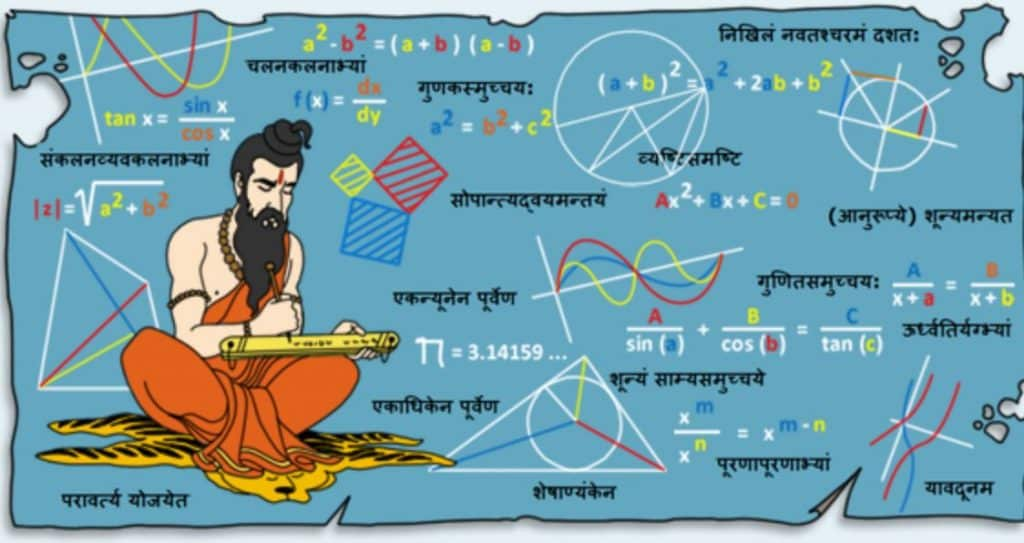In India, mathematics has its roots in the Vedic literature which is nearly 4000 years old. Between 1000 B.C. and 1000 A.D. various concepts on mathematics were given by Indian mathematicians in which were set forth for the first time, the concept of zero, the techniques of algebra and algorithm, square root and cube root. There are various examples of mathematics from ancient India that are applied even today.

### 1. Zero

If it weren’t for Indian mathematician-astronomer Aryabhata, there wouldn’t have been a number zero.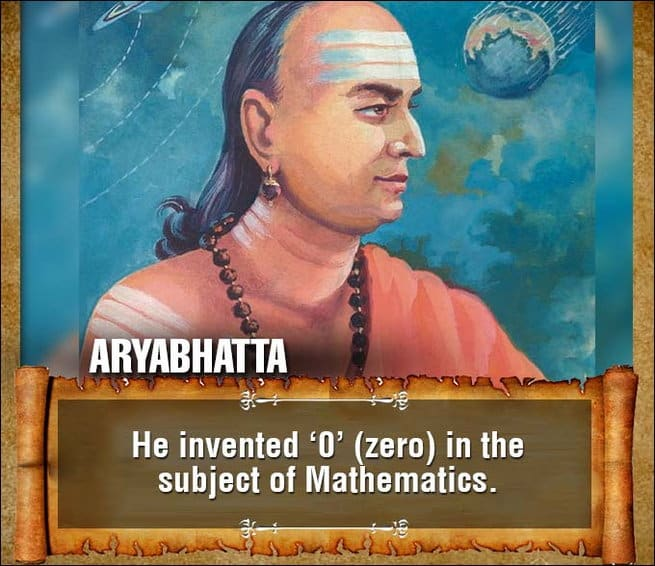Though people have always understood the concept of nothing or having nothing, the concept of zero is relatively new; it fully developed in India around the fifth century A.D. Before then, mathematicians struggled to perform the simplest arithmetic calculations. Today, zero — both as a symbol (or numeral) and a concept meaning the absence of any quantity — allows us to perform calculus, do complicated equations, and to have invented computers.

“The zero is widely seen as one of the greatest innovations in human history, is the cornerstone of modern mathematics and physics, plus the spin-off technology,” said Peter Gobets, secretary of the ZerOrigIndia Foundation, or the Zero Project.

### 2. Algebra

As much as the students hate it today, India has had big contributions in the field of Algebra in ancient times.

In ancient India, conventional mathematics termed Ganitam was known before the development of algebra. This is borne out by the name – Bijaganitam, which was given to the algebraic form of computation. Bijaganitam means ‘the other mathematics’ (Bija means ‘another’ or ‘second’ and Ganitam means mathematics).

In India, around the 5th century A.D., a system of mathematics that made astronomical calculations easy was developed. In those times its application was limited to astronomy as its pioneers were Astronomers. As astronomical calculations are complex and involve many variables that go into the derivation of unknown quantities. Algebra is a short-hand method of calculation and by this feature, it scores over conventional arithmetic.

### 3. Trigonometry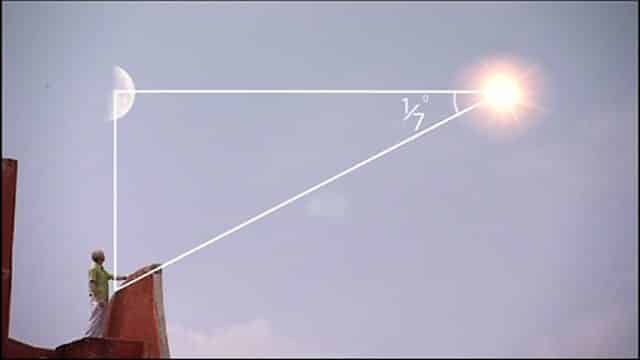Though Trigonometry goes back to the Greek period, the character of the subject started to resemble modern form only after the time of Aryabhata. From here it went to Europe through the Arabs and went into several modifications to reach its present form.  In ancient times Trigonometry was considered a part of astronomy.  Three functions were introduced: jya, kojya and ukramajya.

The first one is r sina where r is the radius of the circle and  sina is the angle subtended at the center.  The second one is r cosa and the third one is r (1 – cosa). By taking the radius of the circle to be 1, we get the modern trigonometric functions. Various relationships between the sine of an arc and its integral and fractional multiples were used to construct sine tables for different arcs lying between 0 and 90°.

### 4. Decimal System and Quadratic formula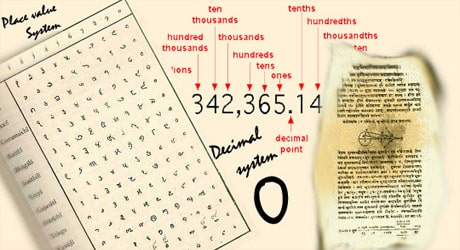In the classical period of Indian mathematics (400 CE to 1600 CE), important contributions were made by scholars like Aryabhata, Brahmagupta, Mahāvīra, Bhaskara II, Madhava of Sangamagrama and Nilakantha Somayaji. The decimal number system in worldwide use today was first recorded in Indian mathematics.

Recommended -  Vaisheshika - Six Different Type of Objects of Experience

It was in 7th century CE when Brahmagupta found the first general formula for solving quadratic equations. The decimal system (or the Hindu number system), which was a precursor of the Arabic numeric system, was developed in India between the 1st and 6th centuries CE.

### 5. Fibonacci Numbers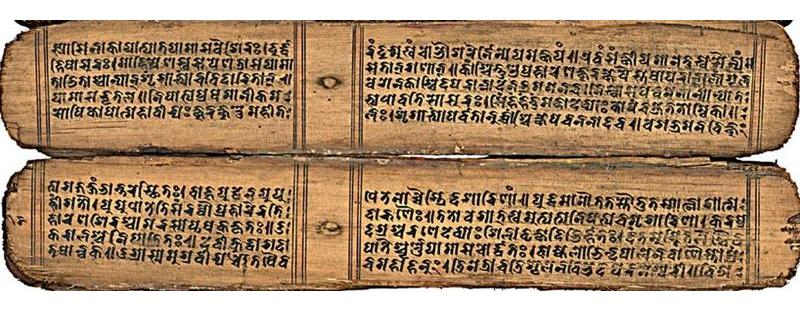The Fibonacci sequence is a series of numbers where a number is found by adding up the two numbers before it. Starting with 0 and 1, the sequence goes 0, 1, 1, 2, 3, 5, 8, 13, 21, 34, and so forth. It was first described by Virahanka, Gopala, and Hemachandra as an outgrowth of earlier writings by Pingala.

### 6. LengthRulers are believed to have been used by the Indus Valley Civilization prior to 1500 BCE. Made of ivory, the rulers found during excavation, reveal the amazing accuracy of decimal subdivisions on it.

The people of the Indus Civilization achieved great accuracy in measuring length, mass, and time. They were among the first to develop a system of uniform weights and measures. A comparison of available objects indicates large scale variation across the Indus territories. Their smallest division, which is marked on an ivory scale found in Lothal in Gujarat, was approximately 1.704 mm, the smallest division ever recorded on a scale of the Bronze Age.

### 7. Weights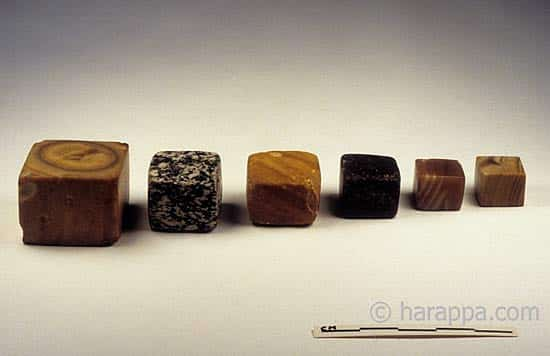The history of measurement systems in India begins in early Indus Valley Civilisation with the earliest surviving samples dated to the 5th millennium BCE. Since early times the adoption of standard weights and measures has reflected in the country’s architectural, folk, and metallurgical artifacts. A complex system of weights and measures was adopted by the Maurya empire (322–185 BCE), which also formulated regulations for the usage of this system. Later, the Mughal Empire (1526–1857) used standard measures to determine land holdings and collect the land tax as a part of Mughal land reforms.

A total of 558 weights were excavated from Mohenjodaro, Harappa, and Chanhu-Daro, not including defective weights. They did not find statistically significant differences between weights that were excavated from five different layers, each measuring about 1.5 m in depth. This was evidence that strong control existed for at least a 500-year period. The 13.7-g weight seems to be one of the units used in the Indus valley. The notation was based on the binary and decimal systems. 83% of the weights which were excavated from the above three cities were cubic, and 68% were made of chert.

### 8. Geometry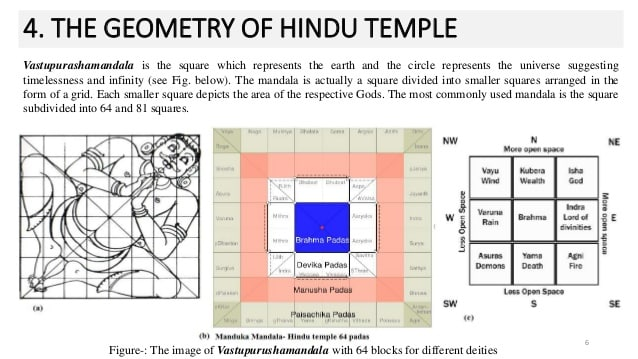Indian mathematicians had their contribution even in the area of Geometry. There was an area of mathematical applications called Rekha Ganita (Line Computation). The Sulva Sutras, which literally mean ‘Rule of the Chord’ give geometrical methods of constructing altars and temples. The temples layouts were called Mandalas. Some of the important works in this field are by Apastamba, Baudhayana, Hiranyakesin, Manava, Varaha, and Vadhula.

### 9. Infinite Series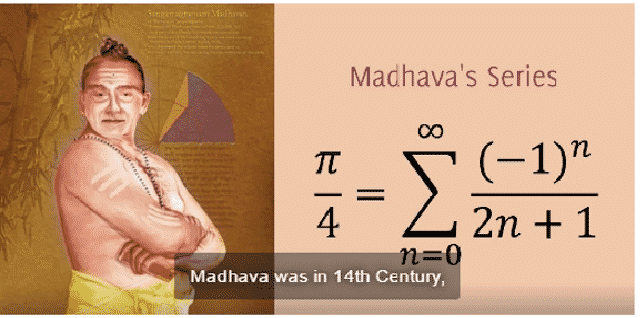Kerala mathematicians produced rules for second-order interpolation to calculate intermediate sine values. The Kerala mathematician Madhava may have discovered the sine and cosine series about three hundred years before Newton.  In this sense, we may consider Madhava to have been the founder of mathematical analysis. Madhava (circa 1340 – 1425 A.D.) was the first to take the decisive step from the finite procedures of ancient Indian mathematics to treat their limit-passage to infinity.

Recommended -  10 Important Life Lessons from Lord Shiva That Everyone Should Learn

His contributions include infinite-series expansions of circular and trigonometric functions and finite-series approximations. His power series for p and for sine and cosine functions is referred to reverentially by later writers.

### 10. Binary CodeBinary numbers form the basis for the operation of computers. Binary numbers were discovered in the west by German mathematician Gottfried Leibniz in 1695. However, new evidence proves that binary numbers were used in India prior to the 2nd century A.D., more than 1500 years before their discovery in the west.

The source of this discovery is a text of music by Pingala named “Chhandahshastra” meaning science of meters. This text falls under the category of “Sutra” or aphorismic statements. Detailed discussions of these short but profound statements are found in later commentaries. “Chhandahshastra” can be conservatively dated to 2nd century A.D. The main commentaries on “Chhandahshastra” are “Vrittaratnakara” by Kedara in probably 8th century, “Tatparyatika” by Trivikrama in the 12th century and “Mritasanjivani” by Halayudha in the 13th century.Subscribe
Notify of